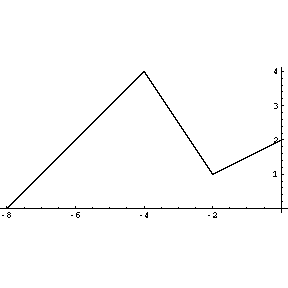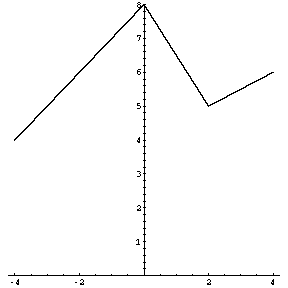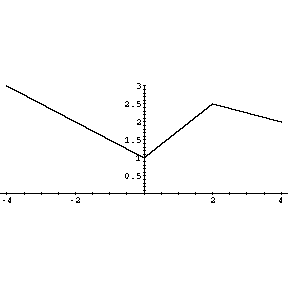``

The graph of fThe graph of f(x+4) is obtained from that of f by means of a horizontal shift through 4 units to the left.The graph of f(x)+4 is obtained from that of f by means of a vertical shift through 4 units upwards.The graph of 2f is obtained from that of f by means of a vertical magnification by factor 2.The graph of -f/2+3 is obtained from that of f by means of a vertical magnification by factor 1/2, followed by a reflection in the x-axis, followed by a vertical shift upwards through 3 units.